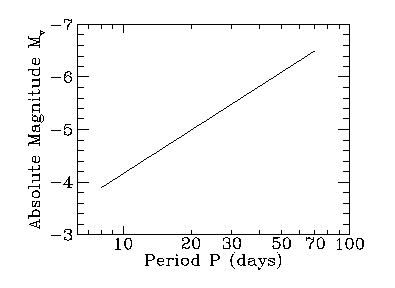### The Period-Luminosity relation

Why are Cepheids so valuable as distance indicators? It is well known that the apparent brightness of an object decreases as the square of its distance. If you know both the apparent and intrinsic brightness of a star, you can calculate how far away it must be. While it is easy to measure the apparent brightness of a star, the intrinsic brightness must be determined indirectly. Cepheids are special in that the period at which Cepheids pulse is related to their average intrinsic luminosity. More luminous Cepheids pulse more slowly. By measuring the period of a Cepheid we can calculate its intrinsic luminosity, and thus its distance.We normally characterize the apparent brightness of a star by its apparent magnitude, mv. The brightest stars in the sky have magnitudes of roughly 0, while the dimmest stars you can see with the unaided eye from a clear, dark location have magnitudes of about 6. Note that brighter stars have smaller magnitudes. Most Cepheids in M100 have apparent magnitudes around 25, which is 40 million times dimmer than the dimmest stars you can see with the unaided eye!

How can we express the intrinsic brightness of a star in terms of magnitudes? One means is to imagine moving the star to a predetermined distance. The absolute magnitude of a star, Mv, is defined to be the apparent brightness of a star when the star is at a distance of 10 pc (32.6 light years). The difference between the apparent magnitude and absolute magnitude provides (almost) enough information to calculate the distance to the star. If you are interested, you can read more about apparent and absolute magnitudes.

In order to find the distance to M100, you need to find the absolute magnitudes for each of the Cepheids. Henrietta Leavitt of the Harvard College Observatory discovered the relationship between the period of Cepheids and their average apparent magnitude mv. She had studied Cepheids in the Large and Small Magellanic Clouds, but did not know the distance to the Cepheids, and therefore could not establish absolute magnitudes or luminosities for the Cepheids. Harlow Shapley determined the calibration needed to turn Leavitt's period - apparent magnitude diagram (P-mv relation) into a period-luminosity relation (P-L relation) for Cepheids. Since the luminosity of a star is related to its absolute visual magnitude (Mv), we can express the P-L relationship as a P-Mv relationship. The P-Mv relationship for M100 is shown graphically below:The relationship is described by the equation (from Ferrarese et al., 1996)

Mv = - [2.76 (log10(P) - 1.0)] - 4.16,

where P is in days. If logarithms are a faint memory, you may wish to peruse a refresher on logs.

For each Cepheid you discovered, use the above equation to determine the absolute magnitude of the Cepheid from its period.

For example, in the demonstration of the Cepheid hunt, you found Cepheid C46. Part I, Section B of your lab sheet for this Cepheid looks like this:

```Cepheid name   Grid #   Avg mv   P(days)    Mv     mv-Mv

C46           47      25.3     25.3
____________   ______   ______   _______  ______  ______
____________   ______   ______   _______  ______  ______
```
From column 4, the period of the Cepheid is 25.3 days. Using the equation above, the average absolute magnitude of C46 is

Mv = -[2.76 (log10(25.3) - 1.0)] - 4.16 = -5.27.

Enter the absolute magnitude in column 5 of Part I, Section B of your lab sheet. The entry for C46 now looks like:

```Cepheid name   Grid #   Avg mv   P(days)    Mv     mv-Mv

C46           47      25.3     25.3    -5.27
____________   ______   ______   _______  ______  ______
____________   ______   ______   _______  ______  ______
```

Find the absolute magnitudes for all your Cepheids, and enter the result in column 5 of Part I, Section B of your lab sheet.

On to The distance to M100

Back to Find the Cepheids!